# Isometric Tiles Math

Working with isometric tiles is a bit trickier than a plain square grid. This tutorial is for beginner game programmers looking to wrap their heads around isometric math. Instead of simply handing you formulas, I intend to explain what they do and how they should be used.

There are many ways of handling isometric tiles but we're only going to talk about one method. This happens to be the most commonly used method. It's the way that isometry is handled in the Tiled map editor, so it's definitely a good approach if you want to use that tool.

## Orthographic Projection

When working in isometric project, your maps will still be a simple 2D array (or equivalent) in memory -- just as if you were working in a simpler orthographic projection (e.g. side or top view).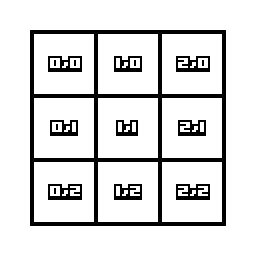Our grid in "map" coordinates -- how our array looks in memory, Values are (map.x, map.y)

If you've worked with a regular square grid before (which I recommend before trying isometric), the math works out pretty simple. Drawing a tile to screen is simply taking the tile's coordinates and multiplying by the tile size to get the screen position:

``` screen.x = map.x * TILE_WIDTH; screen.y = map.y * TILE_HEIGHT; ```

In this example our tiles are 64x64 pixels. If we want to display the tile at position (2,1), we plug the values into our formulae:

``` screen.x = 2 * 64; // equals 128 px screen.y = 1 * 64; // equals 64 px ```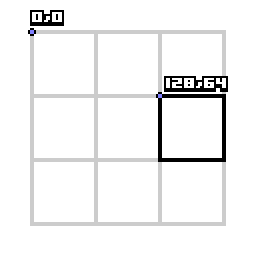Determining the screen position for a tile in Orthographic Projection

## Isometric Projection

This figure shows how we want to project our memory tiles to the screen in Isometric view.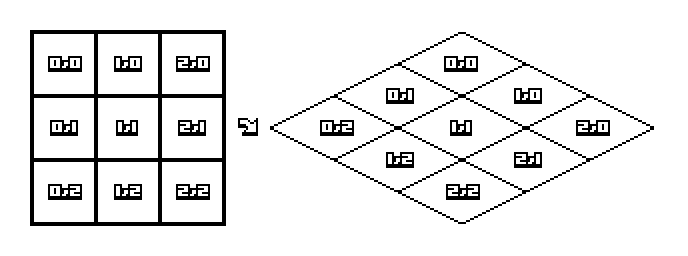We want our map to look like this on the screen

In this example our isometric tiles are 128x64 pixels. Let's draw tile 2,1 again, but this time in isometric projection. First let's measure some pixels to see where tile 2,1 is on the screen compared to the origin point -- so we know what answer we're working towards.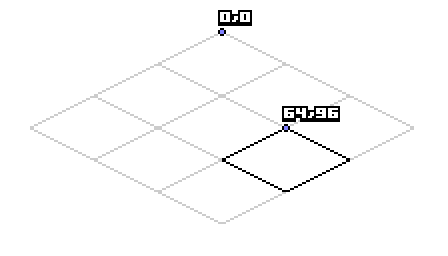Tile 2,1 position in screen pixels is 64,96

Here's where it's easy to get brain-bent. It's possible to calculate rotation and y-scale to do this, but there's a simpler way. The trick is to think of x and y separately. Observe the following about this isometric projection.

Increasing map X by +1 tile (going "right" in map coordinates) increases both screen X and Y (going "right + down" in screen coordinates). If we measure in our example, we'll see that it increases screen.x by 64 (half our tile's width) and screen.y by 32 (half our tile's height).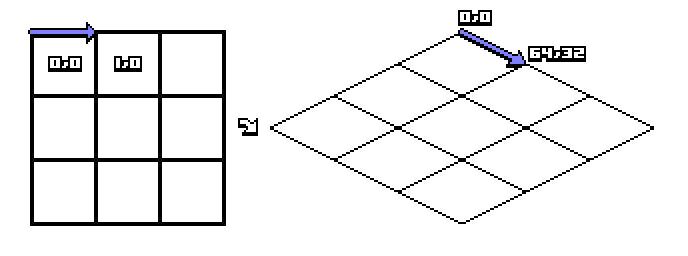map.x++ affects screen pixels by +64,+32

Similarly, increasing map Y by +1 tile (going "down" in map coordinates) decreases screen X and increases screen Y (going "left + down" in screen coordinates).

Expressing those changes as code looks something like this:

``` screen.x = map.x * TILE_WIDTH_HALF - map.y * TILE_WIDTH_HALF; screen.y = map.x * TILE_HEIGHT_HALF + map.y * TILE_HEIGHT_HALF; ```

And with some simplification we get the basic formula for isometric projection:

``` screen.x = (map.x - map.y) * TILE_WIDTH_HALF; screen.y = (map.x + map.y) * TILE_HEIGHT_HALF; ```

Let's test the formula on tile 2,1 to see if we get the expected result:

``` screen.x = (2 - 1) * 64; // equals 64 screen.y = (2 + 1) * 32; // equals 96 ```

## Projecting from Screen pixels back to Map position

Now a square grid is easy to work with. Probably all of your game calculations (e.g. collisions) will happen in square map coordinates. You only project to screen pixels when you need to draw something.

Sometimes though you have to convert screen pixels back to map coordinates. Example: the player clicks on a pixel; how do we reverse the formula and find the tile?

Rather than figure out the formula from the inputs/outputs this time, we're going to use good old Algebra to reverse the functions.

``` // Basic isometric map to screen is: screen.x = (map.x - map.y) * TILE_WIDTH_HALF; screen.y = (map.x + map.y) * TILE_HEIGHT_HALF; // Solve the first equation for map.x screen.x == (map.x - map.y) * TILE_WIDTH_HALF screen.x / TILE_WIDTH_HALF == map.x - map.y map.x == screen.x / TILE_WIDTH_HALF + map.y // Solve the second equation for map.y screen.y == (map.x + map.y) * TILE_HEIGHT_HALF screen.y / TILE_HEIGHT_HALF == map.x + map.y map.y == screen.y / TILE_HEIGHT_HALF - map.x // Replace "map.y" in the first equation with what it equals in the second map.x == screen.x / TILE_WIDTH_HALF + map.y map.x == screen.x / TILE_WIDTH_HALF + screen.y / TILE_HEIGHT_HALF - map.x 2(map.x) == screen.x / TILE_WIDTH_HALF + screen.y / TILE_HEIGHT_HALF map.x == (screen.x / TILE_WIDTH_HALF + screen.y / TILE_HEIGHT_HALF) /2 // And now do the same for map.y map.y == screen.y / TILE_HEIGHT_HALF - (screen.x / TILE_WIDTH_HALF + map.y) map.y == screen.y / TILE_HEIGHT_HALF -(screen.x / TILE_WIDTH_HALF) - map.y 2(map.y) == screen.y / TILE_HEIGHT_HALF -(screen.x / TILE_WIDTH_HALF) map.y == (screen.y / TILE_HEIGHT_HALF -(screen.x / TILE_WIDTH_HALF)) /2 // So final actual commands are: map.x = (screen.x / TILE_WIDTH_HALF + screen.y / TILE_HEIGHT_HALF) /2; map.y = (screen.y / TILE_HEIGHT_HALF -(screen.x / TILE_WIDTH_HALF)) /2; ```Given screen pixel coordinates 64,96, we expect to project back to tile (2,1)

``` map.x = (screen.x / TILE_WIDTH_HALF + screen.y / TILE_HEIGHT_HALF) /2; map.x = (64 / 64 + 96 / 32) /2; map.x = (1 + 3) /2; map.x = 2; map.y = (screen.y / TILE_HEIGHT_HALF -(screen.x / TILE_WIDTH_HALF)) /2; map.y = (96 / 32 - (64 / 64)) /2; map.y = (3 - 1) /2; map.y = 1; ```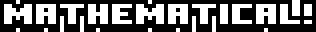## Notes

Notice that the "origin" of the isometric tile is the top corner. But usually when we draw a sprite it's from the top-left corner. Before drawing you may want to adjust by the tile's size. Example for regular sized tiles: screen.x -= TILE_WIDTH_HALF;

These formulas also don't account for a camera. Essentially a camera is a drawing offset, just as in an Orthographic game. The middle of our projected map is at x=0, so if you want it centered on the screen it's like having a camera offset: screen.x += SCREEN_WIDTH_HALF;

Note that these formulas work for sub-tile positions as well. Assuming you're doing all floating-point math, the map position (2.5, 1.5) will become screen position (64.0, 128.0) and vice versa.

If you're going to use floats anyway, you can simplify the screen_to_map functions slightly (because you're not concerned with integer division).

``` // factored out the constant divide-by-two // only if we're doing floating-point division! map.x = screen.x / TILE_WIDTH + screen.y / TILE_HEIGHT; map.y = screen.y / TILE_HEIGHT - screen.x / TILE_WIDTH; ```

I suggest setting up two utility functions for quick conversion between screen and map coordinates. Remember to do all the actual calculations in map coordinates, and only project to/from screen coordinates for inputs (click/touch) and outputs (rendering).

``` Point map_to_screen(Point map_coordinates); Point screen_to_map(Point screen_pixels); ```Clint Bellanger is a software developer who has been experimenting with video game code for 20 years and 3D art for 10 years. His main project is Flare, a Free/Libre action roleplaying engine.